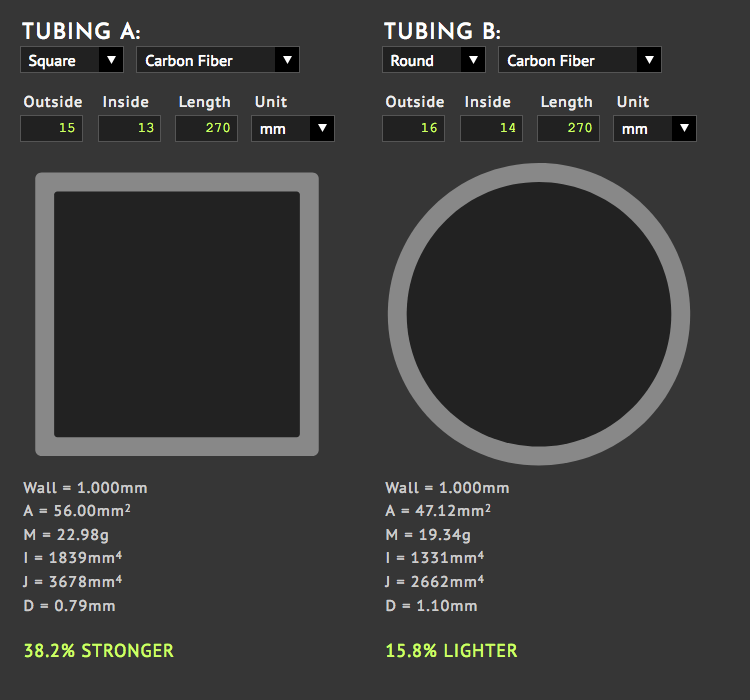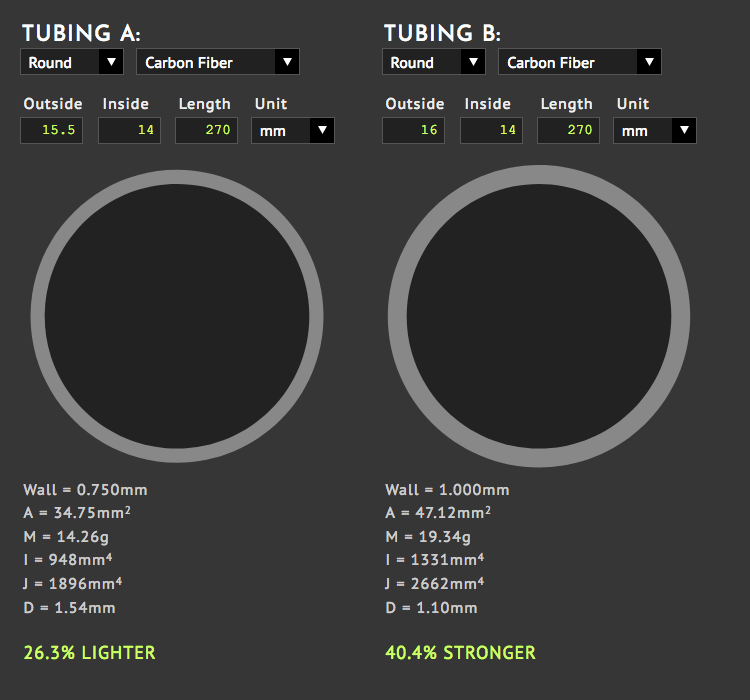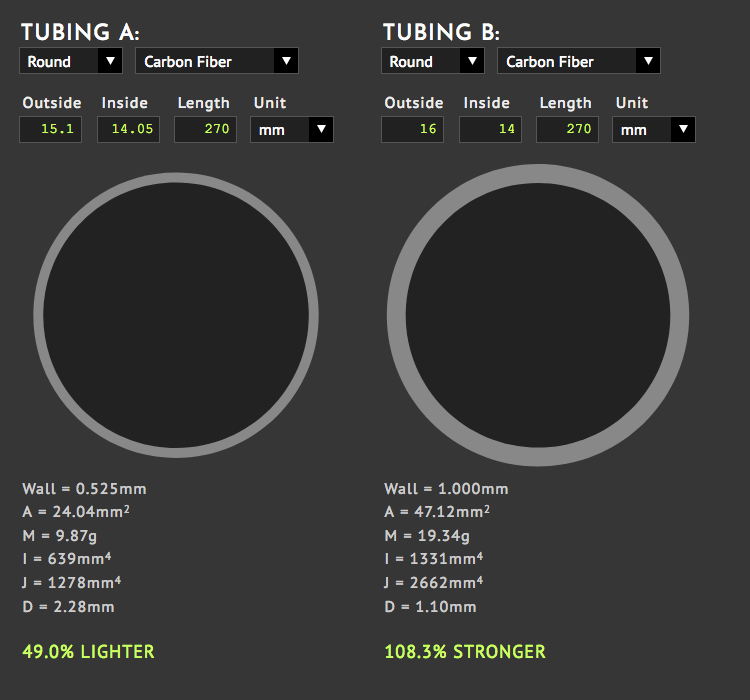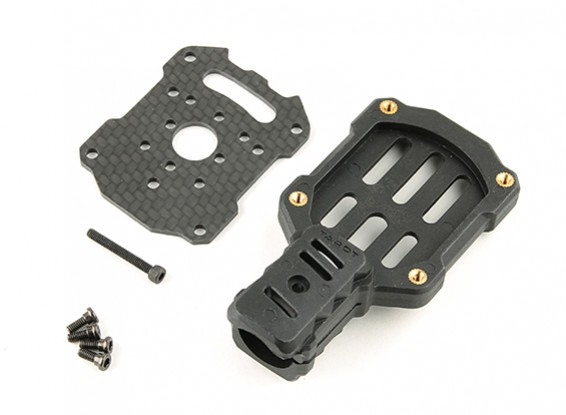# examples

## Tubing Comparison

In this example, we'll compare the weight and strength of several types of tubing, using dCal. All tubing in this example is listed is in the dCal database so the weights were calculated automatically. The weights are all based on 4 pieces of tubing cut to 27cm in length. This is long enough to build a quadcopter with 15" props.

The weights in the dCal database are based on real measurements of actual materials using 2 different scales. The weights generated by the Tubing Calculator are based on average material density. This is why you'll see slightly different numbers in both places.

## Square vs Round

The Tubing Calculator defaults to a comparison of the two most commonly used sizes of tubing for medium sized drones. All we have to do is enter the length and we get:Wall = Wall thickness is shown in the same unit used for input.
A = Cross section area in mm2, inches are converted to millimeters.
M = Mass of cross section area x length. This is ONLY an estimate based on material density. The actual weight of carbon fiber products will vary due to the concentration of resin used in each one.
I = Second Moment of Inertia, Ixx = Iyy for round and square tubing.
J = Polar Moment of Inertia, the ability to resist torsion.
D = Deflection, with one end of the tube fixed and a 2 pound weight (0.9kg) placed on the other end.

Notice the square tubing is stronger but the round tubing is lighter. But also look at calculated values for D, which is the deflection when one of the tube is attached to a wall and a 2 pound weight is placed on the other end. Sure, the square tubing bends less but in this example, its less than 1mm. Weight would seem to be the more important factor here if you're after increased flight time. But read on because there are other factors...

## Dragonplate vs "STANDARD"

Here's the Tubing Calulator chart for the "Standard" 16mm round tubing on the right, with Dragonplate's 15.5mm carbon fiber round tubing on the left:Here, we see a more significant weight savings with the Dragonplate tubing being 26% lighter than the "standard" 16mm tubing from Hobby King and others. Even though the 16mm tubing is 40% stronger, the difference in D (deflection) is still less than 1mm.

Remember to multiply the weights shown in the Tubing Calculator by 4 for a quadcopter, or just use the weights in the dCal PARTS list because they are already multiplied for you.

## Rock West vs "STANDARD"

The next chart compares a 15.1mm carbon fiber tubing from Rock West against the "Standard" 16mm tubing:This Rock West tubing is the lightest 15 to 16mm tubing in the database. As you can see, it is HALF the weight of the standard 16mm tubing used by many drone kits! But is it strong enough? If you look at the D numbers, you can see the Rock West tubing bends twice as much. But that's 2mm over 27cm, with a 2 pound weight hung from the end. Is that acceptable? When designing a drone, we must consider the weight of the motor and prop on the end of the arm, plus the force of the thrust generated while flying.

The Rock West tubing has proven itself in several quadcopter designs that have set records or achieved consistent 40 minute flight times carrying a GoPro and 3D gimbal. Unlike the other tubing in this list, it can snap fairly easily on impact.

## Don't Forget the Motor Mounts

When we're trying to decide which tubing to use, don't forget to include the motor mounts. One popular motor mount is this one from Tarot:These mounts weigh 26g each, or 104g for four. A set of these motor mounts weighs more than any of the sets of tubing!

You could use the square tubing at 82g and bolt the motors directly to the arms. Compare this to 76g for the standard 16mm tubing, plus 104g for the motor mounts, and you have a total of 180g, more than TWICE the weight of using square tubing.

But surely you can find or make lighter motor mounts. How light do they need to be? Lets take the weight of the square tubing, 82g and subtract the lightest tubing from Rock West, 36g. When we subtract 36 from 82, we're left with 46g for motor mounts. That means each mount needs to weigh 11.5g just be the SAME weight as the square tubing! Now compare the strength. Something to think about.

## Parts

Sort by: type, name | weight | created | modified